Chapter 5, Problem 5.6EP### Organic And Biological Chemistry

7th Edition
STOKER + 1 other
ISBN: 9781305081079

#### Solutions

Chapter
Section### Organic And Biological Chemistry

7th Edition
STOKER + 1 other
ISBN: 9781305081079
Textbook Problem

# Write the general structural formula for the functional group present in each of the following carboxylic acid derivatives. a. Amide b. Ester c. Acid anhydride d. Acid chloride

(a)

Interpretation Introduction

Interpretation:

General structural formula of functional group present in amide has to be written.

Concept Introduction:

Carbonyl groups are the one which contain a double bond between carbon and oxygen atom.  Aldehydes and ketones possess this carbonyl functional group in it.  The structural representation of a carbonyl group can be given as shown below,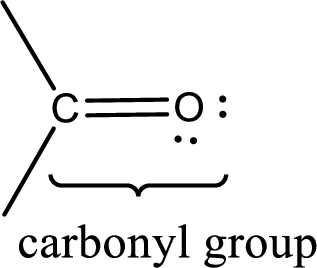If a hydroxyl group is attached to a carbonyl group means it is known as carboxyl group.  This can be represented as shown below,Carboxylic acid derivatives are the ones that are synthesized from or converted to a carboxylic acid.  The generalized structural representation of carboxylic acid derivatives is shown below,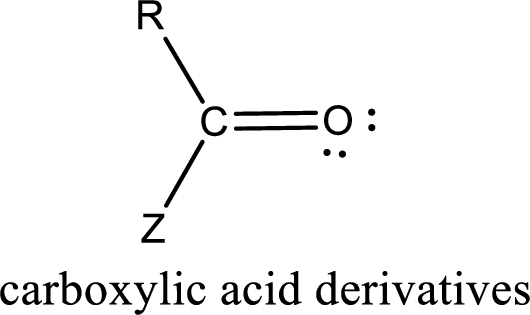Explanation

The general structure of carboxylic acid derivatives is,

The entity Z is not a carbon atom.  For a compound to be amide, “Z” in the above structure has to be a nitrogen atom.  Therefore, the general structure of amide can be represented as shown below,

(b)

Interpretation Introduction

Interpretation:

General structural formula of functional group present in ester has to be written.

Concept Introduction:

Carbonyl groups are the one which contain a double bond between carbon and oxygen atom.  Aldehydes and ketones possess this carbonyl functional group in it.  The structural representation of a carbonyl group can be given as shown below,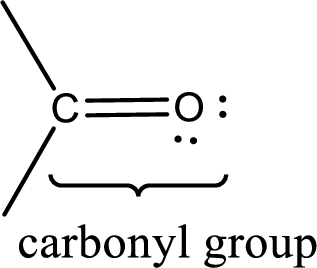If a hydroxyl group is attached to a carbonyl group means it is known as carboxyl group.  This can be represented as shown below,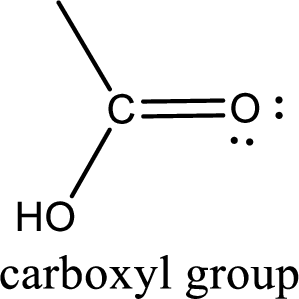Carboxylic acid derivatives are the ones that are synthesized from or converted to a carboxylic acid.  The generalized structural representation of carboxylic acid derivatives is shown below,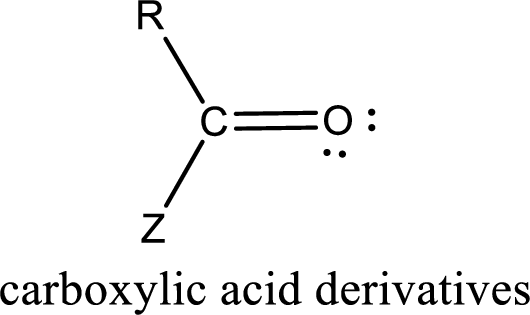(c)

Interpretation Introduction

Interpretation:

General structural formula of functional group present in acid anhydride has to be written.

Concept Introduction:

Carbonyl groups are the one which contain a double bond between carbon and oxygen atom.  Aldehydes and ketones possess this carbonyl functional group in it.  The structural representation of a carbonyl group can be given as shown below,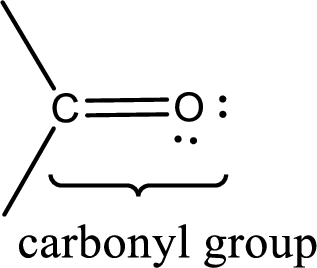If a hydroxyl group is attached to a carbonyl group means it is known as carboxyl group.  This can be represented as shown below,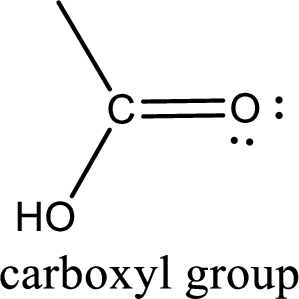Carboxylic acid derivatives are the ones that are synthesized from or converted to a carboxylic acid.  The generalized structural representation of carboxylic acid derivatives is shown below,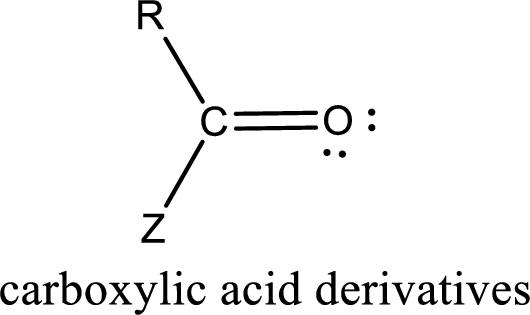(d)

Interpretation Introduction

Interpretation:

General structural formula of functional group present in acid chloride has to be written.

Concept Introduction:

Carbonyl groups are the one which contain a double bond between carbon and oxygen atom.  Aldehydes and ketones possess this carbonyl functional group in it.  The structural representation of a carbonyl group can be given as shown below,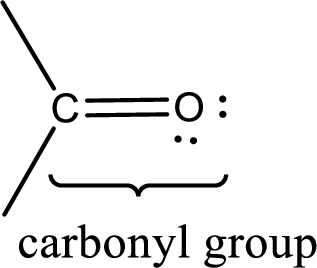If a hydroxyl group is attached to a carbonyl group means it is known as carboxyl group.  This can be represented as shown below,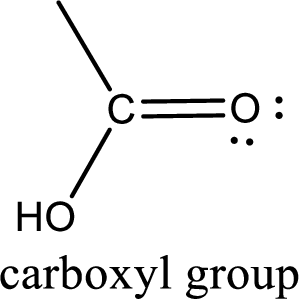Carboxylic acid derivatives are the ones that are synthesized from or converted to a carboxylic acid.  The generalized structural representation of carboxylic acid derivatives is shown below,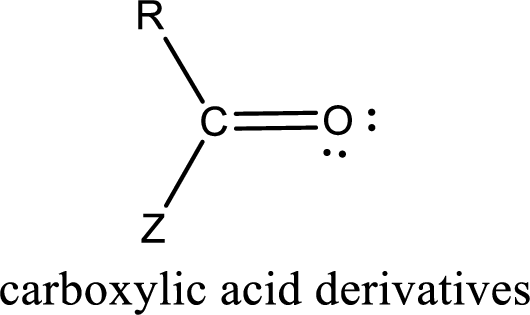### Still sussing out bartleby?

Check out a sample textbook solution.

See a sample solution

#### The Solution to Your Study Problems

Bartleby provides explanations to thousands of textbook problems written by our experts, many with advanced degrees!

Get Started